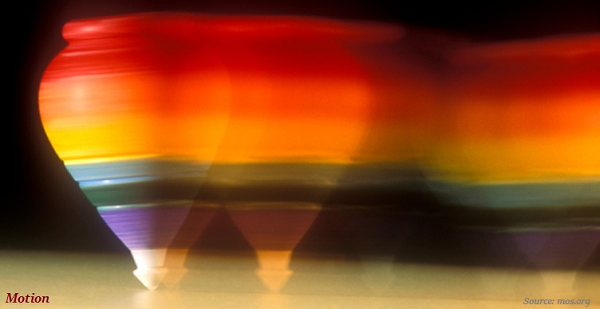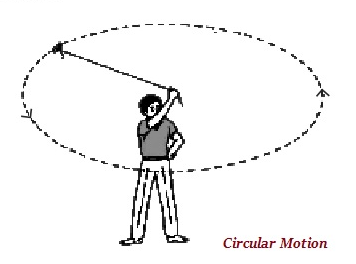# Physics - Motion

## Introduction

• Motion means change in position of an object in given period of time.

• Motion, normally, is described in terms of displacement, velocity, acceleration, distance, time, and speed.## Motion Along a Straight Line

• Motion along a straight line is the simplest form of motion.

• Magnitude is the numerical value of a physical quantity.

• The shortest distance, which is measured from the initial to the final position of an object is called as the ‘displacement.’

• The magnitude of the displacement for a path of motion may be zero but the corresponding distance covered cannot be zero.

• If an object travels equal distances in equal intervals of time, it is said to be in ‘uniform motion.’

• If an object travels unequal distances in equal intervals of time, it is said to be in ‘non-uniform motion.’

## Speed

• The distance travelled by the object in unit time is known as the rate of motion or simply speed.

• The SI unit of speed is meter per second (symbol m s–1 or m/s).

• The average speed of an object can be obtained by dividing the total distance travelled by the total time taken: represented as

$$Average\:Speed = \frac{Total\:Distance\:Travelled}{Total\:Time\:Taken}$$

## Velocity

• If a quantity specifies the direction of motion along with its speed, it is known as velocity.

• Velocity is the speed of a given object, which is moving in a defined direction.

• Speed and velocity have the same measuring units, i.e., m s–1 or m/s.

$$Average\:Velocity = \frac{Initial\:Velocity\:+\:Final\:Velocity}{2}$$

## Acceleration

• The change in the velocity of an object per unit time is defined as acceleration.

• The acceleration is calculated as −

$$Acceleration = \frac{Change\:in\:Velocity}{Time\:Taken}$$

• The SI unit of acceleration is m s–2.

## Uniform Circular Motion

• When an object moves in a circular path in uniform speed, its motion is known as uniform circular motion.• The motion of the earth and all other planets and their satellites is almost in a circular orbit at constant speed.